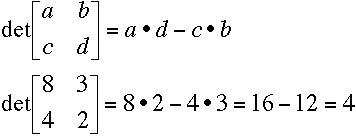Both matrix and determinants are the part of business mathematics. Both are useful for solving business problem. Both are helpful for calculation of each other. For calculation of inverse of matrix, we need to calculate the determinant. For calculating the value of 3X3 matrix or more matrix, we need to divide determinants in sub-matrix.  but there are many differences between matrix and determinants which we can explain in following points.

1. Matrix is the set of numbers which are covered by two brackets. Determinants is also set of numbers but it is covered by two bars.

2. It is not necessary that number of rows will be equal to the number of columns in matrix. But it is necessary that number of rows will be equal to the number of columns in determinant.

3.  Matrix can be used for adding, subtracting and multiplying the coefficients.  Determinant can be used for calculating the value of x,y and z with Cramer's Rule..

## \$type=three\$a=hide\$cm=hide\$s=hide\$show=/2019/06/10-steps-to-become-better-investor.html\$l=hide

Name

false
ltr
item
Svtuition: Difference Between Matrix and Determinant
Difference Between Matrix and Determinant
http://4.bp.blogspot.com/-O_ZhwB6JzVI/VIGIe69CSZI/AAAAAAAAQkQ/PYeW0IpLLyg/s1600/determinant.gif
http://4.bp.blogspot.com/-O_ZhwB6JzVI/VIGIe69CSZI/AAAAAAAAQkQ/PYeW0IpLLyg/s72-c/determinant.gif
Svtuition
http://www.svtuition.com/2012/03/difference-between-matrix-and.html
http://www.svtuition.com/
http://www.svtuition.com/
http://www.svtuition.com/2012/03/difference-between-matrix-and.html
true
3065238051582539150
UTF-8